Geometry

# Regular Polygons Warmup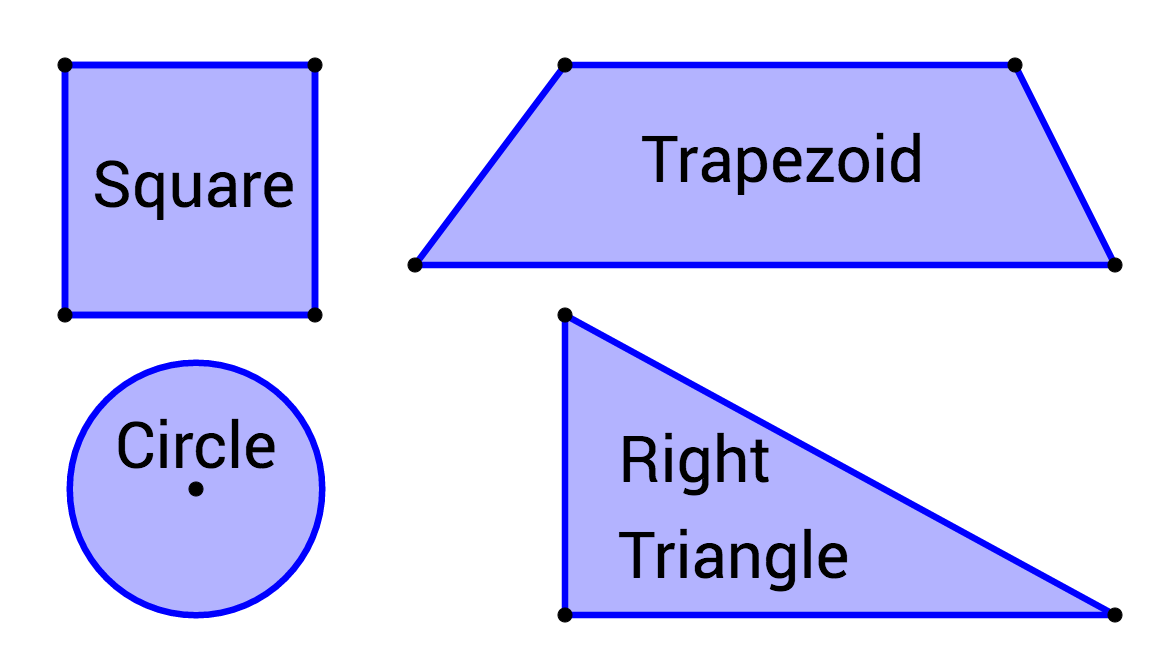Which shape is a regular polygon?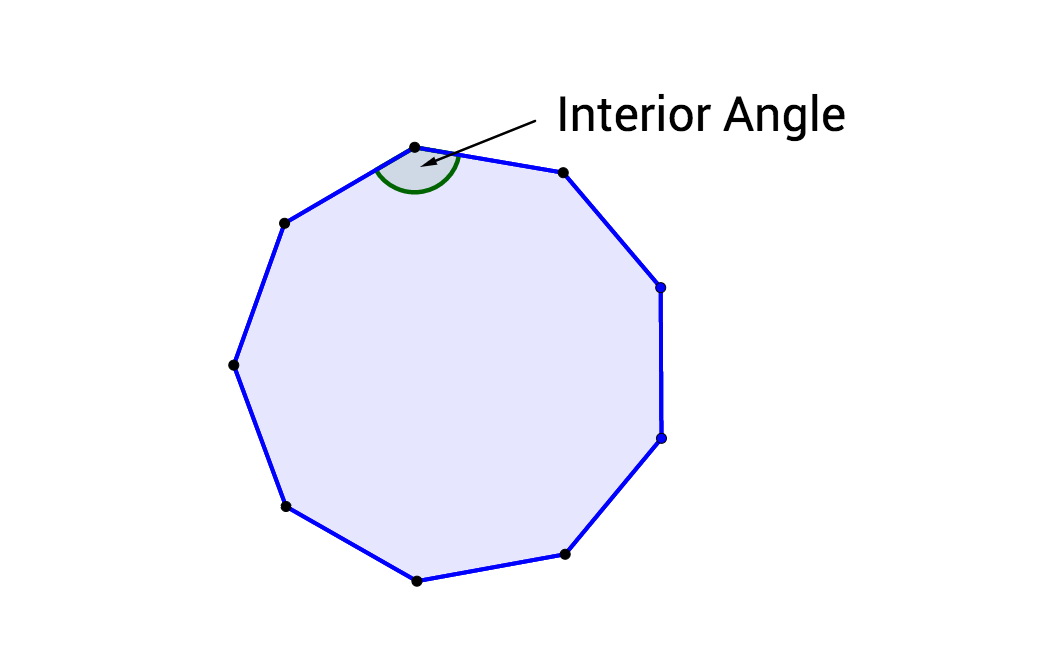If the sum of the interior angles in a polygon equals $1080^\circ,$ how many sides does the polygon have? (Hint: A triangle has an interior angle sum of $180^\circ.$ A quadrilateral has an interior angle sum of $360^\circ.$ A pentagon has an interior angle sum of $540^\circ.$)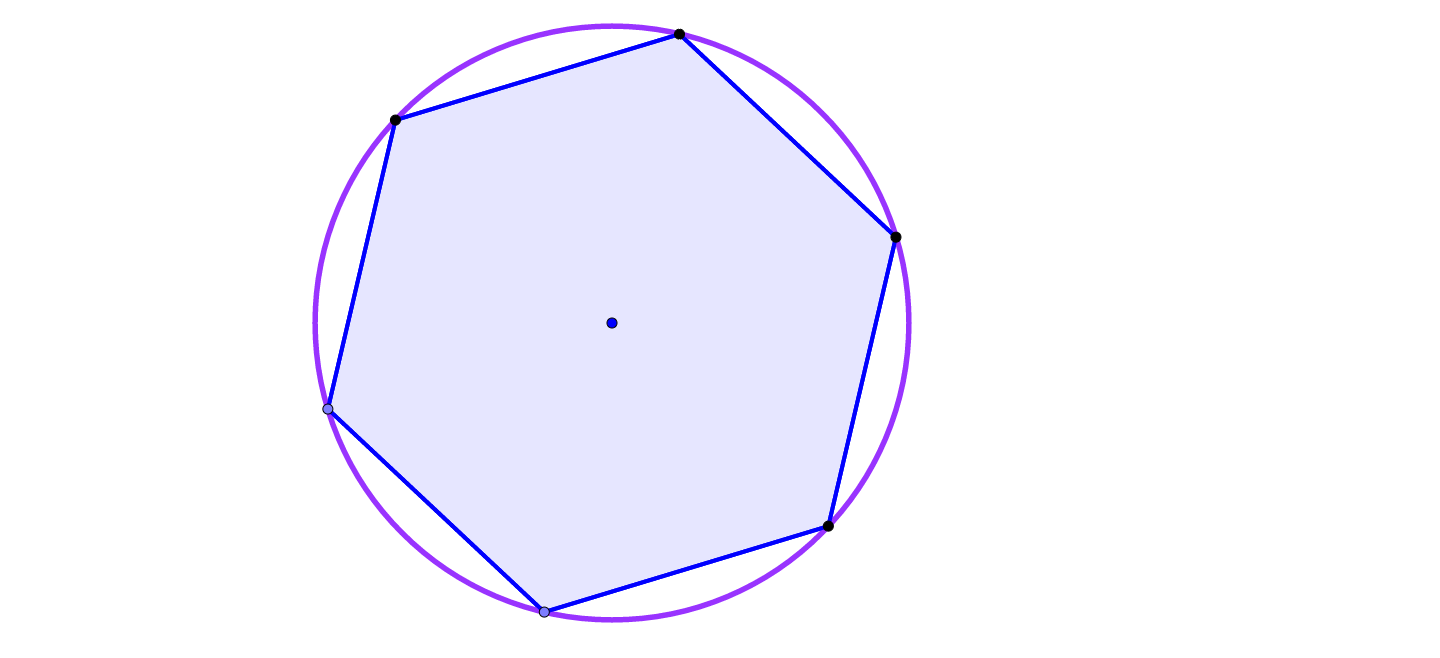A hexagon is inscribed in a circle with a radius of 6. What is the area of the hexagon?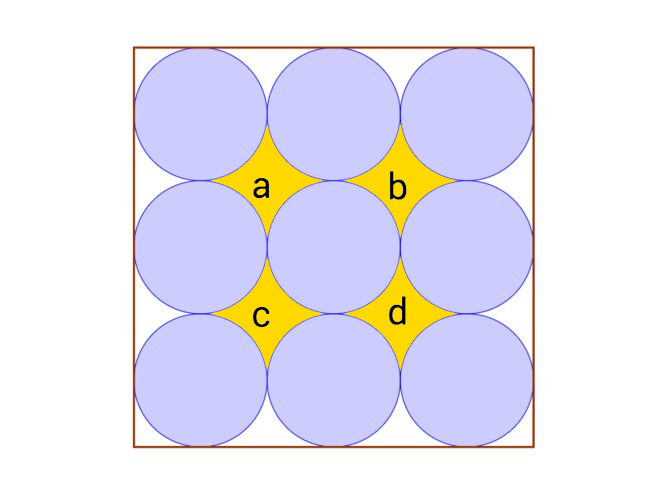Nine identical circles are placed adjacent to one another in three rows in a square whose area is 9. $a, b, c,$ and $d$ represent the areas of those regions between the circles. What is $a + b + c + d?$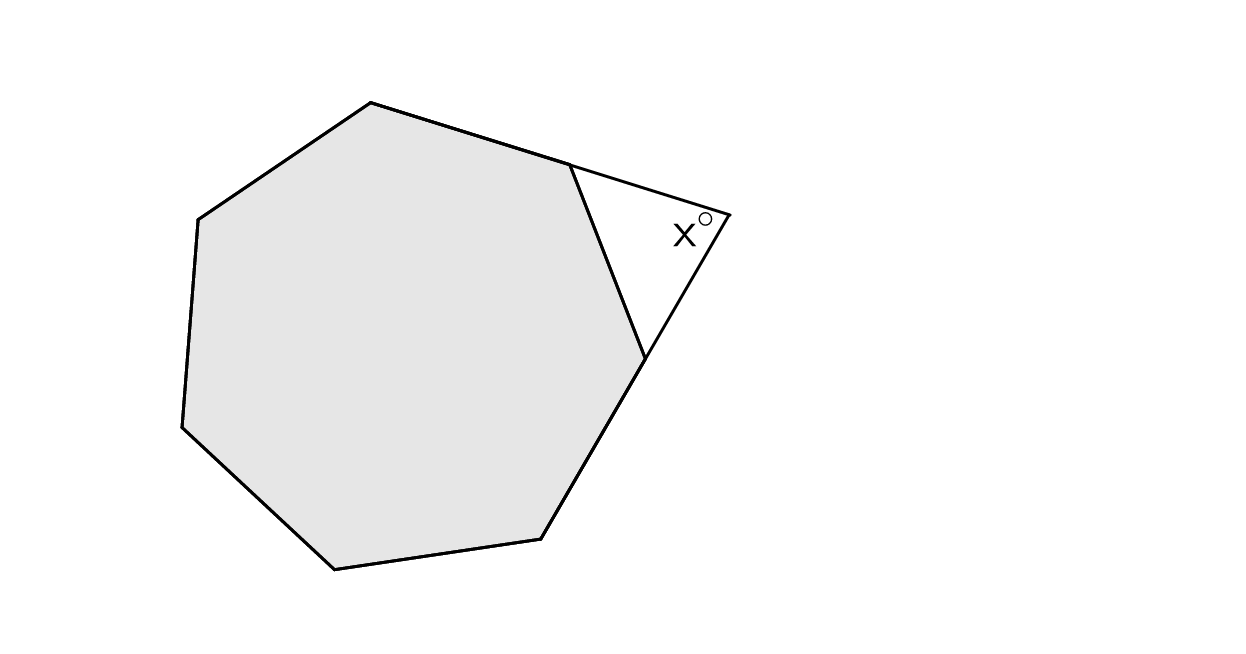An angle is formed by extending two sides of a regular heptagon, as shown. What is the closest approximate value of $x?$

×